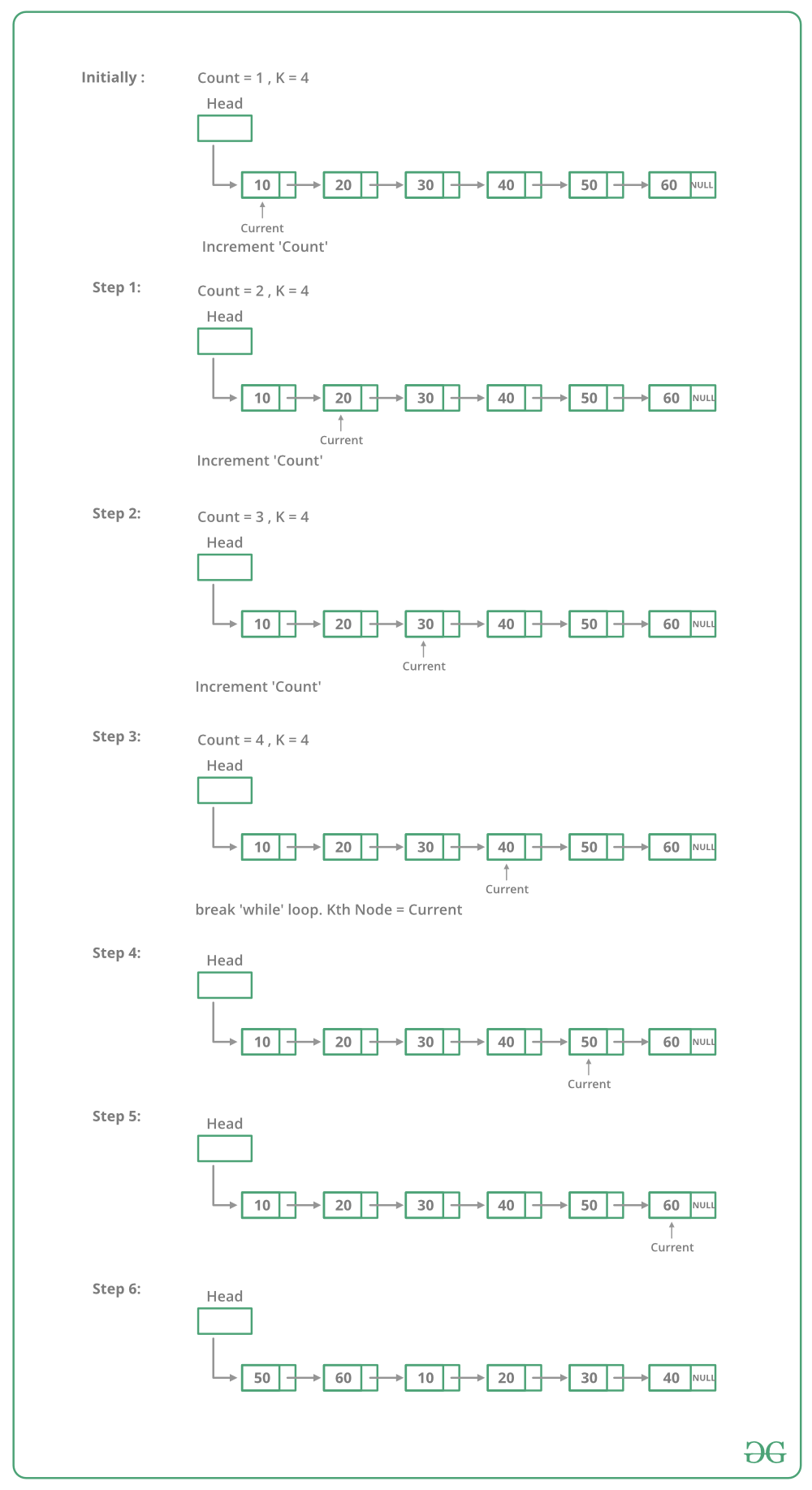GeeksforGeeks App
Open AppBrowser
Continue

# Java Program For Rotating A Linked List

Given a singly linked list, rotate the linked list counter-clockwise by k nodes. Where k is a given positive integer. For example, if the given linked list is 10->20->30->40->50->60 and k is 4, the list should be modified to 50->60->10->20->30->40. Assume that k is smaller than the count of nodes in a linked list.

Method 1:
To rotate the linked list, we need to change the next of kth node to NULL, the next of the last node to the previous head node, and finally, change the head to (k+1)th node. So we need to get hold of three nodes: kth node, (k+1)th node, and last node.
Traverse the list from the beginning and stop at kth node. Store pointer to kth node. We can get (k+1)th node using kthNode->next. Keep traversing till the end and store a pointer to the last node also. Finally, change pointers as stated above.

Below image shows how to rotate function works in the code :## Java

 `// Java program to rotate a``// linked list``class` `LinkedList``{``    ``// Head of list``    ``Node head;` `    ``// Linked list Node``    ``class` `Node``    ``{``        ``int` `data;``        ``Node next;``        ``Node(``int` `d)``        ``{``            ``data = d;``            ``next = ``null``;``        ``}``    ``}` `    ``// This function rotates a linked list``    ``// counter-clockwise and updates the``    ``// head. The function assumes that k is``    ``// smaller than size of linked list. It``    ``// doesn't modify the list if k is greater``    ``// than or equal to size``    ``void` `rotate(``int` `k)``    ``{``        ``if` `(k == ``0``)``            ``return``;` `        ``// Let us understand the below code``        ``// for example k = 4 and list =``        ``// 10->20->30->40->50->60.``        ``Node current = head;` `        ``// current will either point to kth or``        ``// NULL after this loop. current will``        ``// point to node 40 in the above example``        ``int` `count = ``1``;``        ``while` `(count < k && current != ``null``)``        ``{``            ``current = current.next;``            ``count++;``        ``}` `        ``// If current is NULL, k is greater than``        ``// or equal to count of nodes in linked list.``        ``// Don't change the list in this case``        ``if` `(current == ``null``)``            ``return``;` `        ``// current points to kth node. Store it in a``        ``// variable. kthNode points to node 40 in the``        ``// above example``        ``Node kthNode = current;` `        ``// current will point to last node after this``        ``// loop current will point to node 60 in the``        ``// above example``        ``while` `(current.next != ``null``)``            ``current = current.next;` `        ``// Change next of last node to previous head``        ``// Next of 60 is now changed to node 10` `        ``current.next = head;` `        ``// Change head to (k+1)th node``        ``// head is now changed to node 50``        ``head = kthNode.next;` `        ``// change next of kth node to null``        ``kthNode.next = ``null``;``    ``}` `    ``/*  Given a reference (pointer to pointer)``        ``to the head of a list and an int, push``        ``a new node on the front of the list. */``    ``void` `push(``int` `new_data)``    ``{``        ``/* 1 & 2: Allocate the Node &``                  ``Put in the data*/``        ``Node new_node = ``new` `Node(new_data);` `        ``// 3. Make next of new Node as head``        ``new_node.next = head;` `        ``// 4. Move the head to point to``        ``// new Node``        ``head = new_node;``    ``}` `    ``void` `printList()``    ``{``        ``Node temp = head;``        ``while` `(temp != ``null``)``        ``{``            ``System.out.print(temp.data + ``" "``);``            ``temp = temp.next;``        ``}``        ``System.out.println();``    ``}` `    ``// Driver code``    ``public` `static` `void` `main(String args[])``    ``{``        ``LinkedList llist = ``new` `LinkedList();` `        ``// Create a list``        ``// 10->20->30->40->50->60``        ``for` `(``int` `i = ``60``; i >= ``10``; i -= ``10``)``            ``llist.push(i);` `        ``System.out.println(``               ``"Given list"``);``        ``llist.printList();` `        ``llist.rotate(``4``);` `        ``System.out.println(``               ``"Rotated Linked List"``);``        ``llist.printList();``    ``}``}``// This code is contributed by Rajat Mishra`

Output:

```Given linked list
10  20  30  40  50  60
50  60  10  20  30  40```

Time Complexity: O(n) where n is the number of nodes in Linked List. The code traverses the linked list only once.

Auxiliary Space: O(1) as it is using constant space

Method 2:
To rotate a linked list by k, we can first make the linked list circular and then moving k-1 steps forward from head node, making (k-1)th node’s next to null and make kth node as head.

## Java

 `// Java program to rotate a``// linked list counter clock wise``import` `java.util.*;` `class` `GFG{` `// Link list node``static` `class` `Node``{``    ``int` `data;``    ``Node next;``};``static`  `Node head = ``null``;``  ` `// This function rotates a linked list``// counter-clockwise and updates the``// head. The function assumes that k is``// smaller than size of linked list.``static` `void` `rotate( ``int` `k)``{``    ``if` `(k == ``0``)``        ``return``;` `    ``// Let us understand the below``    ``// code for example k = 4 and``    ``// list = 10.20.30.40.50.60.``    ``Node current = head;` `    ``// Traverse till the end.``    ``while` `(current.next != ``null``)``        ``current = current.next;` `    ``current.next = head;``    ``current = head;` `    ``// Traverse the linked list to``    ``// k-1 position which will be``    ``// last element for rotated array.``    ``for` `(``int` `i = ``0``; i < k - ``1``; i++)``        ``current = current.next;` `    ``// Update the head_ref and last``    ``// element pointer to null``    ``head = current.next;``    ``current.next = ``null``;``}` `// UTILITY FUNCTIONS``// Function to push a node``static` `void` `push(``int` `new_data)``{ ``    ``// Allocate node``    ``Node new_node = ``new` `Node();` `    ``// Put in the data``    ``new_node.data = new_data;` `    ``// Link the old list of the``    ``// new node``    ``new_node.next = head;` `    ``// Move the head to point to``    ``// the new node``    ``head = new_node;``}` `// Function to print linked list``static` `void` `printList(Node node)``{``    ``while` `(node != ``null``)``    ``{``        ``System.out.print(node.data + ``" "``);``        ``node = node.next;``    ``}``}` `// Driver code``public` `static` `void`  `main(String[] args)``{``    ``// Start with the empty list``    ``// Create a list 10.20.30.40.50.60``    ``for` `(``int` `i = ``60``; i > ``0``; i -= ``10``)``        ``push(i);` `    ``System.out.print(``           ``"Given linked list "``);``    ``printList(head);``    ``rotate( ``4``);` `    ``System.out.print(``           ``"Rotated Linked list "``);``    ``printList(head);``}``}``// This code is contributed by gauravrajput1`

Output:

```Given linked list
10 20 30 40 50 60